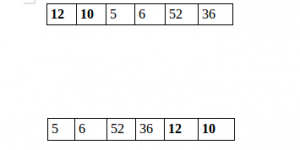GeeksforGeeks App
Open AppBrowser
Continue

# Python Program to Split the array and add the first part to the end

There is a given array and split it from a specified position, and move the first part of the array add to the end.Examples:

```Input : arr[] = {12, 10, 5, 6, 52, 36}
k = 2
Output : arr[] = {5, 6, 52, 36, 12, 10}
Explanation : Split from index 2 and first
part {12, 10} add to the end .```
```Input : arr[] = {3, 1, 2}
k = 1
Output : arr[] = {1, 2, 3}
Explanation : Split from index 1 and first

Method 1:

## Python3

 `# Python program to split array and move first``# part to end.`  `def` `splitArr(arr, n, k):``    ``for` `i ``in` `range``(``0``, k):``        ``x ``=` `arr[``0``]``        ``for` `j ``in` `range``(``0``, n``-``1``):``            ``arr[j] ``=` `arr[j ``+` `1``]` `        ``arr[n``-``1``] ``=` `x`  `# main``arr ``=` `[``12``, ``10``, ``5``, ``6``, ``52``, ``36``]``n ``=` `len``(arr)``position ``=` `2` `splitArr(arr, n, position)` `for` `i ``in` `range``(``0``, n):``    ``print``(arr[i], end``=``' '``)` `# Code Contributed by Mohit Gupta_OMG <(0_o)>`

Output

`5 6 52 36 12 10 `

Time complexity: O(nk), where n is the length of the array and k is the number of times the first part of the array needs to be moved to the end.
Auxiliary space: O(1), the program only uses a constant amount of additional memory.

Method 2:

## Python3

 `# Python program to split array and move first``# part to end.`  `def` `splitArr(a, n, k):``    ``b ``=` `a[:k]``    ``return` `(a[k::]``+``b[::])`  `# main``arr ``=` `[``12``, ``10``, ``5``, ``6``, ``52``, ``36``]``n ``=` `len``(arr)``position ``=` `2``arr ``=` `splitArr(arr, n, position)``for` `i ``in` `range``(``0``, n):``    ``print``(arr[i], end``=``' '``)`

Output

`5 6 52 36 12 10 `

Time Complexity: O(n), where n is the length of the input array ‘arr’.
Auxiliary Space: O(k), where k is the value of ‘position’ input parameter.

Method  3: Using slicing and extend() methods

## Python3

 `# Python program to split array and move first``# part to end.` `arr ``=` `[``12``, ``10``, ``5``, ``6``, ``52``, ``36``]``n ``=` `len``(arr)``position ``=` `2``x ``=` `arr[:position]``y ``=` `arr[position:]``y.extend(x)``for` `i ``in` `y:``    ``print``(i, end``=``" "``)`

Output

`5 6 52 36 12 10 `

Time complexity: O(n)
Auxiliary space: O(n)

Method  4: Using list comprehension and modulo:

Another approach is to split an array into two parts and add the first part to the end of the second part. This operation is commonly used in programming and can be useful for a variety of applications, such as rotating the elements of an array or implementing circular buffers.

• Define the function split_and_add(arr, n) that takes an array arr and an integer n as input.
• Compute the length of the input array using the len function and store it in a variable arr_len.
• Use a list comprehension to create a new list result of the same length as the input array, where each element of the new list is computed using the formula (i + n) % arr_len where i is the index of the current element in the input array.
• Return the new list result.

## Python3

 `def` `split_and_add(arr, n):``    ``return` `[arr[(i ``+` `n) ``%` `len``(arr)] ``for` `i ``in` `range``(``len``(arr))]`  `arr ``=` `[``12``, ``10``, ``5``, ``6``, ``52``, ``36``]``n ``=` `2` `result ``=` `split_and_add(arr, n)` `print``(``*``result)`

Output

`5 6 52 36 12 10`

Time complexity: O(n) : where n is length of the input array. This is because we use a list comprehension to create a new list, and this operation takes O(n) time. The modulo operation % takes constant time, so it does not contribute to the overall time complexity.
Auxiliary space: O(n) : we create a new list of the same length as the input array. This list is used to store the results of the computation, so it takes O(n) space in memory.

Please refer complete article on Split the array and add the first part to the end for more details!

Method  5: Using deque module from the collections:

Algorithm:

1. Import the deque module from the collections package.
2. Define a function splitArr() that takes an array a, its length n, and an integer k as input.
3. Create a deque object q from the input array a using the deque() constructor.
4. Rotate the deque object q by -k positions using the rotate() method. This operation effectively moves the first k elements of the array to the end of the deque, and shifts the remaining elements to the front.
5. Convert the deque object q back to a list using the list() constructor and return the result.
In the main program, initialize an array arr, its length n, and a position variable k.
Call the splitArr() function with the input array arr, length n, and position k as arguments.
Iterate over the resulting array and print each element.

## Python3

 `from` `collections ``import` `deque` `def` `splitArr(a, n, k):``  ``q ``=` `deque(a)``  ``q.rotate(``-``k)``  ``return` `list``(q)` `# main``arr ``=` `[``12``, ``10``, ``5``, ``6``, ``52``, ``36``]``n ``=` `len``(arr)``position ``=` `2``arr ``=` `splitArr(arr, n, position)``for` `i ``in` `range``(``0``, n):``  ``print``(arr[i], end``=``' '``)``  ``#This code is contributed by Jyothi pinjala.`

Output

`5 6 52 36 12 10 `

Time complexity: O(n) since it rotates the deque with k elements, which takes O(k) time, and then returns the list, which takes O(n) time. Since k is constant, the time complexity is linear with respect to n.
Auxiliary Space: O(n), since it creates a deque and a list, each with n elements. The space used by the deque and the list are both proportional to the size of the input array, so the space complexity is linear with respect to n.

My Personal Notes arrow_drop_up